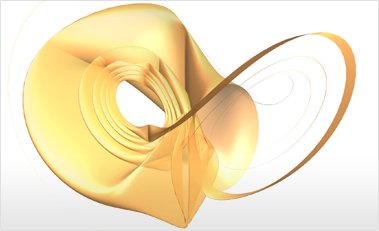# Parametric Differential Equations

Mathematica 9 leverages the extensive numerical differential equation solving capabilities of Mathematica to provide functions that make working with parametric differential equations conceptually simple. New algorithms have been developed to compute derivatives of arbitrary target functions via sensitivity solutions.• Handles parameters anywhere in the problem specification.
• Solutions given as ParametricFunction objects that evaluate with specific parameter values.
• Derivatives of ParametricFunction objects automatically computed using algorithms for sensitivity solutions.
• Ability to compute functional of differential equation solution directly.
• Automatic caching of solutions speeds up computations.
• Support for ODEs, DAEs, DDEs, and PDEs with parameters.
• Makes it easy to perform parameter sweeps.
• Makes it easy to search for and optimize parameter values in differential equations.
• Makes it easy to fit differential equations to data, i.e. calibration.

## Mathematica

Questions? Comments? Contact a Wolfram expert »